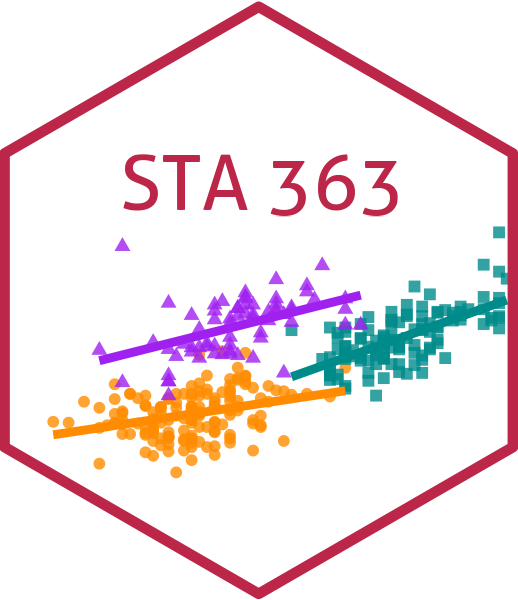# Ensemble Models

## Ensemble Models

Let’s stack models together!

## Ensemble Models

• Take your output from tune_grid for several different model types
• “Stack” them together into a single prediction model
• The single model uses Lasso to predict the outcome using the predictions from the other models
• You get a coefficient that you then apply for each candidate model to get a final prediction!

## So how do we do it?

• As I mentioned, we can just use our output from tune_grid with one tiny addition – we need to tell R that we want to save a bit more information in each of the tuned models so that we can smoosh them all together
library(tidymodels)
ctrl <- control_resamples(
save_pred = TRUE,
save_workflow = TRUE
)
mod <- tune_grid(
wf,
cv,
control = ctrl
)

## Example

library(ISLR)
set.seed(1)
Hitters <- drop_na(Hitters)
cv <- vfold_cv(Hitters)
rec <- recipe(Salary ~ ., data = Hitters) |>
step_dummy(all_nominal_predictors()) |>
step_normalize(all_predictors())

bag_spec <- rand_forest(
mode = "regression",
mtry = ncol(Hitters) - 1,
trees = tune()
)

rf_spec <- rand_forest(
mode = "regression",
mtry = 3,
trees = tune()
)

grid = data.frame(trees = c(10, 50, 100, 1000))

wf_bag <- workflow() |>

wf_rf <- wf_bag |>
update_model(rf_spec)

tune_bag <- tune_grid(wf_bag, cv, grid = grid, control = ctrl)
tune_rf <- tune_grid(wf_rf, cv, grid = grid, control = ctrl)

## Example

library(stacks)
ens <- stacks() |>

ens
# A data stack with 2 model definitions and 8 candidate members:
#   bag: 4 model configurations
#   rf: 4 model configurations
# Outcome: Salary (numeric)

## Example

library(stacks)
ens <- stacks() |>
blend_predictions() |>
fit_members()

ens
# A tibble: 3 × 3
member  type        weight
<chr>   <chr>        <dbl>
1 rf_1_4  rand_forest  0.557
2 bag_1_3 rand_forest  0.326
3 rf_1_2  rand_forest  0.167
collect_parameters(ens, "rf")
# A tibble: 4 × 3
member trees  coef
<chr>  <dbl> <dbl>
1 rf_1_1    10 0
2 rf_1_2    50 0.167
3 rf_1_3   100 0
4 rf_1_4  1000 0.557

## Example

preds <- ens |>
predict(new_data = Hitters, members = TRUE) |>
bind_cols(Hitters |> select(Salary))

map_dfr(preds, rmse, truth = Salary, data = preds) |>
mutate(member = colnames(preds))
# A tibble: 5 × 4
.metric .estimator .estimate member
<chr>   <chr>          <dbl> <chr>
1 rmse    standard        114. .pred
2 rmse    standard        115. bag_1_3
3 rmse    standard        127. rf_1_2
4 rmse    standard        124. rf_1_4
5 rmse    standard          0  Salary